# Non Verbal Reasoning - Rule Detection

Exercise : Rule Detection - Section 1
Directions to Solve

In each of the following questions, choose the set of figures which follows the given rule.

1.

Choose the set of figures which follows the given rule.

Rule: Closed figures losing their sides and open figures gaining their sides.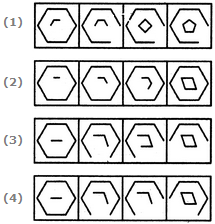1
2
3
4
Explanation:
No answer description is available. Let's discuss.

2.

Choose the set of figures which follows the given rule.

Rule: Any figure can be traced by a single unbroken line without retracting.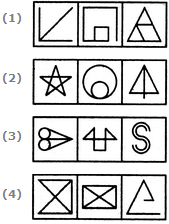1
2
3
4
Explanation:
No answer description is available. Let's discuss.

3.

Choose the set of figures which follows the given rule.

Rule: Closed figures gradually become open and open figures gradually become closed.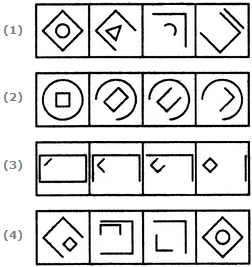1
2
3
4
Explanation:
No answer description is available. Let's discuss.

4.

Choose the set of figures which follows the given rule.

Rule: Closed figures become more and more open and open figures become more and more closed.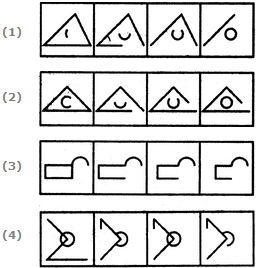1
2
3
4
Explanation:
No answer description is available. Let's discuss.

5.

Choose the set of figures which follows the given rule.

Rule: The series becomes complex as it proceeds.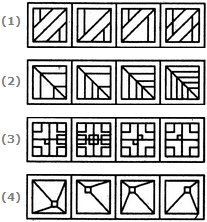1
2
3
4